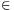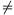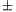Mathematical and Physical Journal
for High Schools
Issued by the MATFUND Foundation
 Already signed up? New to KöMaL?

#Problem A. 378. (September 2005)

A. 378. Does there exist a functionsuch that f(x)=-f(y) whenever x and y are different rationals and xy=1 or x+y{0,1}?

(5 pont)

Deadline expired on October 17, 2005.

Solution. There exists such a function.

Consider the continued fraction form of the rational numbers. As is well-known, each rational x can be uniquely written aswhere a0 is an integer, a1,...,an are positive integers and an>1.

For an arbitrary rational x, lettx be the number of divisions in the continued fraction form of x (the number n above). Then(x)=0, for inegers and(x)=(1/x)+1 for all 0<x<1.

Now define function f. Set

f(0)=-1;for all x>0;, for all x<0.

By the definition of(x) we have that for all positive rational x and positive integer k,(x+k)=(x) and f(x+k)=f(x).

Now prove f(1/x)=-f(x), if x0,1. Since f(-x)=-f(x) and f(-1/x)=-f(1/x), it can assumed that x>0. Moreover, the roles of x and 1/x are symmetric so we can assume 0<x<1. Then(x)=(1/x)+1, therefore f(1/x)=-f(x).

From the definition of f we know that f(x)=-f(y) for all x+y=0, except x=y=0. The final property which must be verified is that f(1-x)=-f(x) if. By the symmetry assume.

If x>1 then f(x)=f(x-1)=-f(1-x), done.

If x=1 then f(x)=f(1)=1 and f(1-x)=f(0)=-1, done.

The only remaining case is when. thenTherefore, function f satisfies all the required properties.

Remark. It is easy to see that there exist exactly two solutions: f and -f.

### Statistics:

 23 students sent a solution. 5 points: Erdélyi Márton, Gyenizse Gergő, Hujter Bálint, Jankó Zsuzsanna, Kisfaludi-Bak Sándor, Kónya 495 Gábor, Molnár 999 András, Nagy 224 Csaba, Paulin Roland, Sümegi Károly, Szabó 108 Tamás, Ureczky Bálint. 4 points: Korándi Dániel, Radnai András, Szilágyi Dániel. 3 points: 2 students. 2 points: 3 students. 0 point: 3 students.

Problems in Mathematics of KöMaL, September 2005